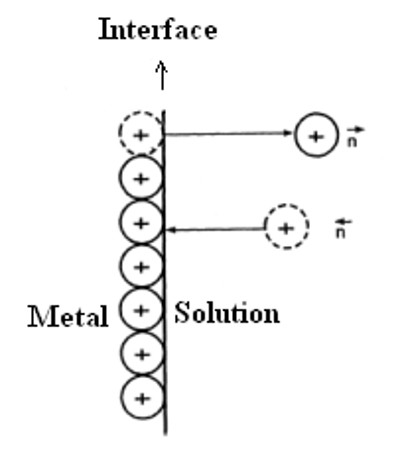# Origin of E.M.F. at Metal Solution Interface

The origin of E.M.F. at metal Solution Interface

Mention has been made of the potential difference between the electrodes. The question one may ask is: what is meant by the term potential of the electrode and how does the potential difference arise?

The origin of the potential difference between the two electrodes may be traced to the relative tendencies of zinc and copper, or any other metal to go into solution as ions. Zinc has a greater tendency to form ions than copper; that is why this familiar fact that when a zinc rod is dipped into a copper sulphate solution a deposit of copper metal is obtained. Nernst supposed that whenever an electrode is immersed into an electrolytic solution containing its ions (say copper electrode dipped into a solution containing Cu2+ ions), there is a tendency of the electrode metal to go into solution as ions. This is opposed by the positive charge on the ions.

Nernst equation relates the equilibrium potential of an half cell with the standard electrode potential, temperature, activity and reaction quotients of the reacting species. It is named after the German physical chemist Walther Nernst.As the concentration of the metal ions in solution increases this opposition increases and the reverse process of deposition of positive ions on the electrode starts taking place. An equilibrium is finally reached when the rate at which the ions are formed is equal to the rate at which the ions are deposited. The equilibrium can be represented as:

Mn+ (aq) + ne ↔ M (s)

A potential develops at the interface between the solid electrode and the electrolyte solution. This potential is called electrode potential. The electrode potential indicates the tendency of an electrode either to lose or gain electrons in the electrode reactions. More precisely, it is the potential difference of the electrical double layer formed at the contact interface of electrode metal and the electrolytic solution. It is also called single electrode potential. From the above kinetic viewpoint Nernst derived the relation

E = E0 + RT/nF ln [Mn+]

for the electrode potential, E. Here [Mn+] is the concentration of the ion of valence n, T is the temperature in K, R is the universal gas constant and F is the Faraday. E°, which is called the standard electrode potential, is the value of E when [Mn+] is unity. So one can say that-

The standard potential of an electrode is the potential difference between a metal and its ions in solution of concentration one mol L-1.

Standard electrode potentials are used to compare the tendency of the metal to lose electrons or gain electrons.sup